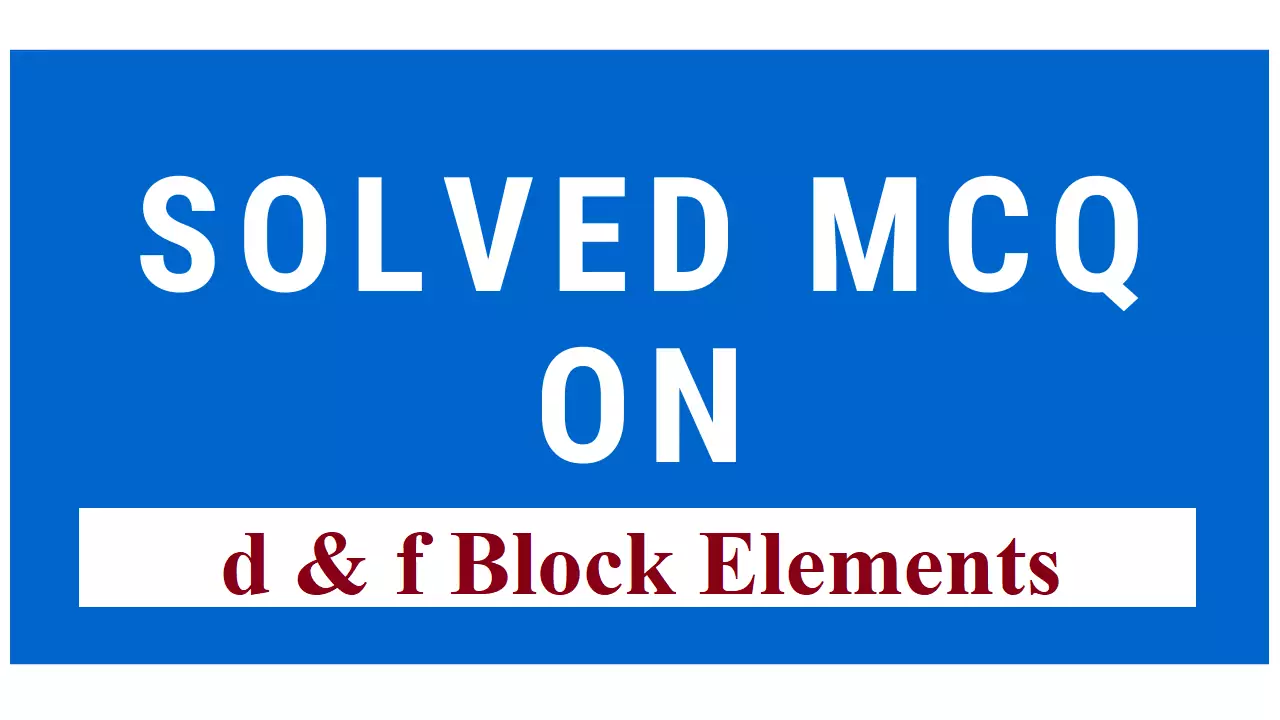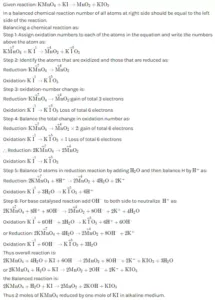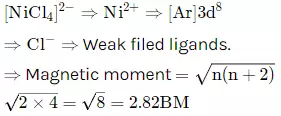# Solved MCQs d & f Block ElementsSolved MCQs d & f Block Elements

Solved MCQs d & f Block Elements:

Que 1. Four successive members of the first-row transition elements are listed below with their atomic numbers. Which one of them is expected to have the highest third ionisation enthalpy?
b) Chromium (Z = 24)
c) Manganese (Z = 25)
d) Iron (Z = 26)
Ans 1. (c) It can be seen after losing 2 electrons, Mn has 3d5 configuration which is exactly half filled. More energy is required to remove the third electron.

Que 2. The aqueous solution containing which one of the following ions will be colourless?
a) Sc3+                 b) Fe2+                      c) Ti3+                    d) Mn2+ (Atomic number: Sc = 21, Fe = 26, Ti = 22, Mn = 25)
Ans 2. (a) Sc3+ has d0 configuration thus its aqueous solution is colourless. Only those salts give colour in aqueous solution whose ions contain unpaired electrons in the d-subshell.

Que 3. Which one of the following elements with the following outer orbital configuration may exhibit the largest number of oxidation states?
a) 3d2 4s2
b) 3d34s2
c) 3d54d1
d) 3d54s2
Ans 3. (d) In the transition elements, the energies of (n-1)d orbitals and ns orbitals are very close. Hence, electrons from both can participate in bonding. Hence option (4) will have maximum oxidation states.

Que 4. Which one of the following ions has electronic configuration [Ar] 3d6?
a) Co3+
b) Ni3+
c) Mn3+
d) Fe3+
Ans 4. (a) The outer configuration are: Co: 3d7 4s2
Ni: 3d8 4s2
Mn: 3d5 4s2
Fe: 3d4 4s2
To form tripositive ion, each atom has to lose three electrons each. Thus, the given configuration matches with that of Co3+ ion.

Que 5. Transition elements have the maximum tendency to form complexes because
a) they are metals and all metals form complexes
b) they contain incompletely filled d-orbitals
c) their charge/size ratio is quite large
d) of both (b) and (c)
Ans 5. (d) of both (b) and (c)

Que 6. In a transition series, as the atomic number increases, paramagnetism
c) first increases to a maximum and then decreases
d) first decreases to a minimum and then increases
Ans 6. c (first increases to a maximum and then decreases)

Que 7. Which of the following transition metal ions will have a definite value of magnetic moment?
a) Sc3+
b) Ti3+
c) Cu+
d) Zn2+
Ans 7. b (Ti3+)

Que 8. Cuprous ion is colourless, while cupric ion is coloured because
a) both have unpaired electrons in the d-orbital
b) cuprous ion has a complete d-orbital and cupric ion has an incomplete d-orbital
c) both have half-filled p- and d-orbitals
d) cuprous ion has incomplete d-orbital and cupric ion has a complete d-orbital
Ans 8. (b) (cuprous ion has a complete d-orbital and cupric ion has an incomplete d-orbital)

Que 9. Amongst TiF62-, CoF63-, Cu2Cl2 and NiCl42- (At. Nos. Ti = 22, Co = 27, Cu = 29, Ni = 28). The colourless species are
a) CoF63- and NiCl42-
b) TiF62- and CoF63-
c) Cu2Cl2 and NiCl42-
d) TiF62- and Cu2Cl2
Ans 9. (d) (TiF62- and Cu2Cl2)

Que 10. The magnetic moment of a transition metal of 3d-series is 6.92 B.M. Its electronic configuration would be
a) 3d5 4s2
b) 3d5 4s1
c) 3d6 4s0
d) 3d5 4s0
Ans 10. (b) (b. 3d5 4s1)

Que 11. The first ionization enthalpy of elements of 5d-series is higher than those of 3d- and 4dseries. This is because
a) the atomic radii of elements of 5d-series are smaller than those of 3d- and 4d-series
b) the nuclear charges of elements of 5d-series are higher than those of 3d- and 4d-series
c) the valence shell electrons of 5d elements experience greater effective nuclear charge than 3d- and 4d-elements due to poor shielding of 4f subshell electrons
d) there is appreciable shielding of 4f-subshell electrons on valence shell electrons of 5d elements.
Ans 11. (c) (the valence shell electrons of 5d elements experience greater effective nuclear charge than 3d- and 4d-elements due to poor shielding of 4f subshell electrons).

Que 12. Which one of the following shows the highest magnetic moment?
a) V3+
b) Cr3+
c) Fe3+
d) Co3+
Ans 12. (c) (Fe3+)

Que 13. The transition elements are more metallic than the representative elements because they have
a) the electrons in d-orbitals
b) electron pairs in d-orbitals
c) availability of d-orbitals for bonding
d) unpaired electrons in metallic orbitals
Ans 13. (c) (availability of d-orbitals for bonding)

Que 14. Colour in transition metal compounds is attributed to
a) small-sized metal ions
b) absorption of light in the UV region
c) complete ns subshell
d) incomplete (n-1) d subshell
Ans 14. (d) (incomplete (n-1) d subshell)

Que 15. Among the following outermost configurations of transition metals which shows the highest oxidation state?
a) 3d34s
b) 3d54s1
c) 3d34s
d) 3d64s2
Ans 15. (c) (3d34s2)

Que 16. In which of the following ions, d-d transition is not possible?
a) Ti4+
b) Cr3+
c) Mn2+
d) Cu2+
Ans 16. (a) (Ti4+)

Solved MCQs d & f Block Elements

Que 17. The electronic configuration of gadolinium (At. No. = 64)
a) 4f85d06s2
b) 4f75d16s2
c) 4f35d56s2
d) 4f65d26s2
Ans 17. (b) 4f75d16s2

Que 18. Which one of the following elements shows maximum no. of different oxidation states in its compounds?
a) Eu
b) La
c) Gd
d) Am
Ans 18. (d) Am

Que 19. Lanthanoid contraction is due to an increase in:
a) Effective shielding of 4f electrons
b) Atomic no
c) Effective nuclear charge
Ans 19. (c) Effective nuclear charge

Que 20. Which of the following oxidation state is most common among the lanthanoid is:
a) +3
b) +4
c) +2
d) +5
Ans 20. (a) +3

Que 21. The product of oxidation of I with MnO4–   in alkaline medium is
a) IO3
b) I2
c) IO
d) IO4
Ans 21. (a) IO3
Oxidation of   with KMnO4​ is given by,Reaction:
MnO4+ I → IO3 ​+ Mn+2
Product form is ​.

Que 22. The formula of pyrolusite is
a) Mn3O4
b) MnO2
c) MnO
d) Mn2O7
Ans 22. (b) MnO2

Que 23. Which of the following statements is not correct?
a) La(OH)3 is less basic than Lu(OH)3
b) La is actually an element of transition series rather than lanthanoids
c) Atomic radius of Zr and Hf is same
d) In lanthanoid series the ionic radius of Lu3+is smallest
Ans 23. (a) La(OH)3 is less basic than Lu(OH)3

Que 24. The basic character of the transition metal monoxide follows the order
a) CrO > VO > FeO > TiO
b) TiO > FeO > VO > CrO
c) TiO > VO > CrO > FeO
d) VO > CrO > TiO > FeO
Ans 24. (c) TiO > VO > CrO > FeO. As we move across the period, the size of the atom gets reduced because of increasing effective nuclear charge. So, it will be less easy for the elements on the rightmost end of the d-block to show electropositive character because of increasing effective nuclear charge the outer shell es will be more tightly bound to the nucleus. So, the basic character decreases as we move across a period because of a decrease in electropositive character.

Que 25. Which of the following ion has the electronic configuration is 3d6
a) Ni3+
b) Mn3+
c) Fe3+
d) Co3+
Ans 25. (d) Co3+

Que 26. The colour of light absorbed by an aqueous solution of CuSO4
a) Orange-red
b) Blue-green
c) Yellow
d) Violet
Ans 26. (a) Orange-red. The aqueous solution of  is blue in colour because of the absorption of orange-red light absorbed from the visible wavelength of light. Higher the concentration, deeper is the blue colour.

Que 27. Which one of the following statements is correct when SO2 is passed through acidified K2Cr2O7 solution?
a) SO2 is reduced
b) Green Cr2(SO4)3 is formed
c) The solution turns blue
d) The solution is Decolourised
Ans 27. (b) The solution is Decolourised. When ​ is passed through acidified ​Cr2​O7​ solution, green ​(SO4​)3​ is formed.
K2Cr2​O7​ + 3SO2​ + H2​SO4 ​→ K2​SO4​ + Cr2​(SO4​)3​ + H2​O
Sulphur dioxide is oxidized to sulphuric acid. Potassium dichromate is reduced to chromic sulphate. The orange colour of solution turns green.

Que 28. Which of the following statement about the interstitial compound is incorrect?
a) They retain metallic conductivity
b) They are chemically reactive
c) They are much harder than pure metal
d) They have a higher melting point than pure metal
Ans 28. (b) They are chemically reactive.

Que 29. Acidified K2Cr2O7 solution turns green when Na2SO3 is added to it. This is due to the formation of
a) CrSO4
b) Cr2(SO4)3
c) CrO42-
d) Cr2(SO3)3
Ans 29. (b) Cr2(SO4)3
K2​Cr2​O7​ + 3Na2​SO3​ + 4H2​SO4​ → Cr2​(SO4​)3​ +K2​SO4 ​+ 3Na2​SO4​ + 4H2​O
Que 30. In acidic medium, the equivalent wt. of K2Cr2O7 is expressed in term of molecular wt.
a) M
b) M/2
c) M/3
d) M/6
Ans 30. (d) M/6.  Potassium dichromate in acidic medium is a strong oxidizer. It means it gains electrons during a redox reaction. Potassium dichromate in acidic solution results in
K2Cr2​O7​ + 14H+ + 6e− → 2K+ + 2Cr3+ + 7H2​O
There is an overall gain of 6 electrons. Therefore, the equivalent weight is .

Que 31. In acidic medium one mole of MnO4 accept how many moles of electrons in a redox reaction
a) 1
b) 3
c) 5
d) 6
Ans 31. (c) 5. MnO4 ​+ 8H+ + 5e− →Mn2+ + 4H2​O

Que 32. In dichromate dianion
a) 4 Cr-O bonds are equivalent
b) 6 Cr-O bonds are equivalent
c) All Cr-O bonds are equivalent
d) All Cr-O bonds are non-equivalent
Ans 32. (b). The dichromate ion consists of two tetrahedral sharing oxygen at the common corner. The six terminal bonds have the same bond length due to resonance.

Que 33. Among the following transition metal, the maximum number of oxidation state is exhibited by
a) Mn (z=25)
b) Fe (z=26)
c) Cr (z=26)
d) Ti (z=22)
Ans 33. (a). Mn

Que 34. The no of moles of KMnO4 that will be needed to react with one mole of iodide ion in alkaline solution is
a) 2
b) 2/5
c) 1/5
d) 1
Ans 34. (a)Que 35. Name the gas that decolourises acidified KMnO4
a) CO2
b) NO2
c) P2O5
d) SO2
Ans 35. (d). SO2​ readily decolourises acidified solution.  has a purple colour. Sulphur dioxide gas acts as a reducing agent whereas  is an oxidizing agent and gets decolourized.

Que 36. Of the following outer electronic configurations of first transition series atoms, the highest oxidation state is achieved by which one of them.
a) (n-1)d8 ns2
b) (n-1)d5 ns2
c) (n-1)d3 ns2
d) (n-1)d5 ns1
Ans 36. (b) (n-1)d5 ns2

Que 37. The magnetic moment of [NiCl4]2- is
a) 1.82 BM
b) 5.46 BM
c) 2.82 BM
d) 1.41 BM
Ans 37. (c)Que 38. Which of the following pair have the same size
a) Fe2+, Ni2+
b) Zr4+, Ti4+
c) Zr4+, Hf4+
d) Zn2+, Hf4+
Ans 38. (c). Zr4+, Hf4+

Solved MCQs d & f Block Elements